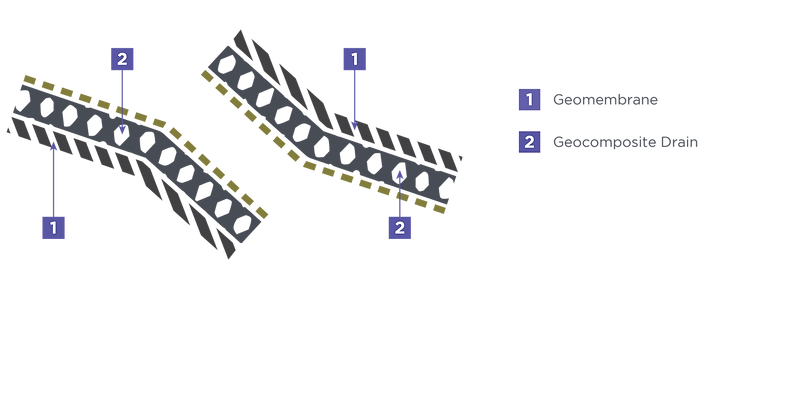# DESIGN METHODS & CONCEPTS

The primary purpose of the geocomposite in a leachate collection system (LCS) is to provide in-plane transmission of leachate that reaches the geocomposite after permeating through the overlaying waste and protective soil cover. The function of the LCS is to convey leachate while controlling the head on the underlying geomembrane. The maximum head must be smaller than the thickness of the drainage system and must be smaller than the required maximum value, typically 0.3 m (1 ft).  Since the geocomposite is commonly designed to convey flow in an unconfined condition (i.e., the liquids are completely maintained within the composite), the buildup of the head is very small.

In a typical landfill cell configuration, the LCS consists of two sections with two different slopes: a steeper upstream side slope; and a flat downstream cell slope.  Figure 5.1 shows a typical cross-section of a landfill leachate collection system with two slopes:FIGURE 5.1 – TYPICAL LANDFILL LEACHATE COLLECTION AND REMOVAL SYSTEM CONFIGURATION

The required transmissivity for geocomposite in the upstream and downstream section can be calculated as follows:

EQUATION 5.1

# down_req

EQUATION 5.2

WHERE

θ            = Design required transmissivity at upstream section

θ                 = Design required transmissivity at downstream section

L      = Length of the liquid collection layer in upstream section

L           = Length of the liquid collection layer in downstream section

β      = Slope for the upstream section

β          = Slope for the downstream section

# down

The ultimate transmissivity for the two section can be calculated using equation (5.3) and (5.4)

EQUATION 5.3

# down_ult               down_req                     in                    cr                    cc                    bc

EQUATION 5.4

WHERE

θ            =

Specified 100-hour transmissivity of a candidate geocomposite layer at upstream section

θ                 =

Specified 100-hour transmissivity of a candidate geocomposite layer at downstream section

RF      = Reduction factor of intrusion

RF      = Reduction factor of creep

RF      = Reduction factor of chemical clogging

RF      = Reduction factor of biological clogging

FS = Overall factor of safety

# EQUATION SHEET

Input Parameter Values

Upstream

Downstream

## Overall Factor of Safety

SOLUTION (Upstream)

## Specified 100hr transmissivity of a candidate geocomposite layer at upstream section

SOLUTION (Downstream)